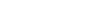# Converting 4-20ma raw values

I’m successfully using a PR33-15 to read data from a flow sensor and I’m generally happy with it. However, I’d like to understand how best to calculate the relationship between the raw values and the current.

All of the code examples I can find contain magic-numbers, either hard-coded raw values or scaling factors. Sometimes a slightly different value is used for each channel. Are these magic numbers obtained by experiment with a 4-20ma signal generator or is it possible to calculate the values using the resolution+gain and information about the components on the board?

Hi,
Current = input voltage * 0.00401
ohm’s law – V = I*R
I = V/R – in this case R is 249

The voltage will depend on gain and vref ( 2.048V).

Voltage = (raw_adc/32767) * (2.048/Gain) * 5.45( op-amp gain)

Thanks

Awesome! Thanks for the reply.